# CIELab Analyses

#### 2021-03-19

Most of the worked examples in the colordistance vignettes use RGB space for simplicity and speed. As discussed in the color spaces vignette, however, you’ll often want to use CIELab color space when possible. This vignette will work through an entire CIELab analysis on the Heliconius butterfly example that comes with the package.

## Choosing a reference white

The convertColor function in the grDevices package allows RGB pixels to be approximately converted into CIELab color space using reference white. The reference white provides information about how a highly reflective (white) surface would look under these lighting conditions, which then allows other colors to be interpreted relative to that white. In the “Luminance” (L) axis of CIELab space, this white would be the 100 on the 0-100 scale.

(A note on this from Ocean Optics: “There is no bound of 100 anywhere. In other words, ‘100 for the brightest white’ is all relative unless you mean ‘the brightest white that is possible in the entire universe’.”)

The supported standard CIE reference whites are:

• A: Standard incandescent bulb.
• B & C: Daylight approximations; deprecated in favor of the more accurate “D” series.
• D50: Direct sunlight.
• D65: Indirect sunlight (most common).

This example will use the “D65” reference white, since it’s usually the default for color conversion.

## Converting from RGB to CIELab

The loadImage function will convert pixels to CIELab space if the CIELab flag is set to TRUE. Because the RGB to CIELab conversion is so slow, colordistance functions that perform CIELab conversions default to converting a random subset of pixels, specified with the sample.size argument.

# Get a list of all the images in the examples folder
image_folders <- dir(system.file("extdata/Heliconius", package = "colordistance"), full.names = T)
image_paths <- sapply(image_folders, colordistance::getImagePaths)
dim(image_paths) <- NULL

# Read in the first image with CIELab pixels
H1 <- colordistance::loadImage(image_paths, lower = rep(0.8, 3), upper = rep(1, 3),
CIELab = TRUE, ref.white = "D65", sample.size = 10000)
#> From reference white:
#> To reference white: D65
#> Converting 10000 randomly selected pixel(s) from sRGB color space to Lab color space

The pixels are stored in the filtered.lab.2d element:

head(H1\$filtered.lab.2d)
#>          L         a        b
#> 1 27.73446  6.411141 24.68598
#> 2 66.38269 33.340728 62.82853
#> 3 51.66477 21.495618 56.11462
#> 4 16.18667  4.851556 19.17128
#> 5 12.66847  4.605913 15.18030
#> 6 68.67481 15.344993 58.28112

They can be visualized using the plotPixels function, specifying color.space = "lab":

colordistance::plotPixels(H1, lower = rep(0.8, 3), upper = rep(1, 1),
color.space = "lab", ref.white = "D65",
main = "CIELab color space",
ylim = c(-100, 100), zlim = c(0, 100))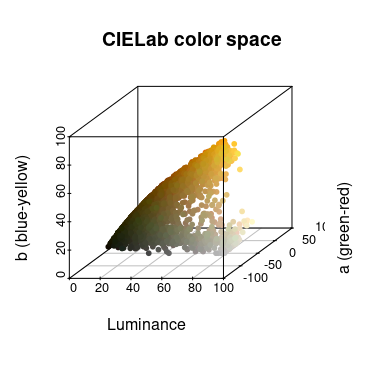## Clustering in CIELab space

Like RGB and HSV, colordistance provides both a histogram binning method and a K-means clustering method for grouping colors together. Note that the histogram binning method uses a different function, getLabHist rather than getImageHist.

The major differences with getLabHist are that it requires the specification of a reference white, and that it gives you the option of setting the ranges for the a and b channels of CIELab space. Unlike RGB, where each channel invariably ranges from 0 to 1, the a and b channels of CIELab are theoretically unbounded. They are usually between -128 and 127, but depending on the reference white may have much narrower ranges than this. There’s no real harm in having boundaries that fall well outside the actual range of the data, but it does have a small impact on both speed and precision.

par(mfrow = c(1, 2))
# Setting boundaries
lab_hist <- colordistance::getLabHist(image_paths, bins = 3,
sample.size = 10000, ref.white = "D65", bin.avg = TRUE,
plotting = TRUE, lower = rep(0.8, 3), upper = rep(1, 3),
a.bounds = c(-100, 100), b.bounds = c(-100, 100))
#> Accuracy of CIE Lab color space depends on specification of an appropriate white reference. See 'Color spaces' vignette for more information.
#> From reference white:
#> To reference white: D65
#> Converting 10000 randomly selected pixel(s) from sRGB color space to Lab color space
#> Using 3^3 = 27 total bins
# Leaving default boundaries (minor difference)
lab_hist <- colordistance::getLabHist(image_paths, bins = 3,
sample.size = 10000, ref.white = "D65", bin.avg = TRUE,
plotting = TRUE, lower = rep(0.8, 3), upper = rep(1, 3))
#> Accuracy of CIE Lab color space depends on specification of an appropriate white reference. See 'Color spaces' vignette for more information.
#> From reference white:
#> To reference white: D65
#> Converting 10000 randomly selected pixel(s) from sRGB color space to Lab color space
#> Using 3^3 = 27 total bins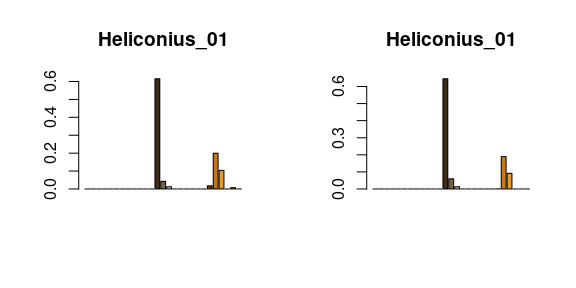getKMeanClusters works almost exactly the same:

lab_kmeans <- colordistance::getKMeanColors(image_paths, n = 2, sample.size = 10000,
lower = rep(0.8, 3), upper = rep(1, 3),
color.space = "CIELab", ref.white = "D65")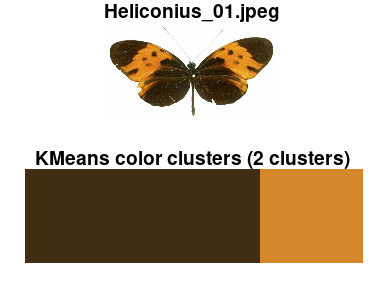## Distance matrices

Once you have CIELab clusters, everything proceeds more or less the same as with RGB color space.

# Generate clusters
par(mfrow = c(2, 4))
lab_hist_list <- colordistance::getLabHistList(image_paths, bins = 2, sample.size = 10000,
ref.white = "D65", lower = rep(0.8, 3), upper = rep(1, 3),
plotting = TRUE, pausing = FALSE)
#> Accuracy of CIE Lab color space depends on specification of an appropriate white reference. See 'Color spaces' vignette for more information.
#> Using 2^3 = 8 total bins
#>
|
|                                                                      |   0%
#>
|
|=========                                                             |  12%
#>
|
|==================                                                    |  25%
#>
|
|==========================                                            |  38%
#>
|
|===================================                                   |  50%
#>
|
|============================================                          |  62%
#>
|
|====================================================                  |  75%
#>
|
|=============================================================         |  88%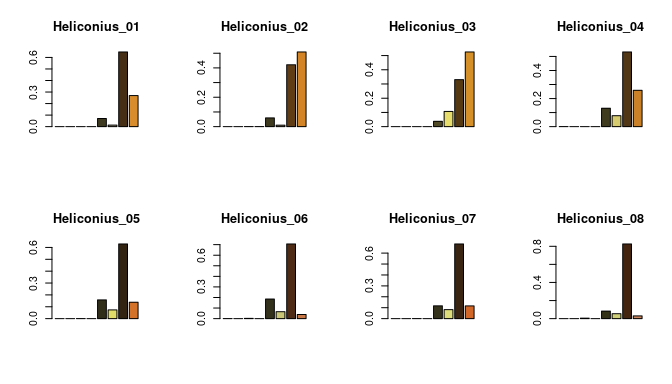#>
|
|======================================================================| 100%
# Get distance matrix
par(mfrow = c(1,1))
lab_dist_matrix <- colordistance::getColorDistanceMatrix(lab_hist_list, plotting = TRUE)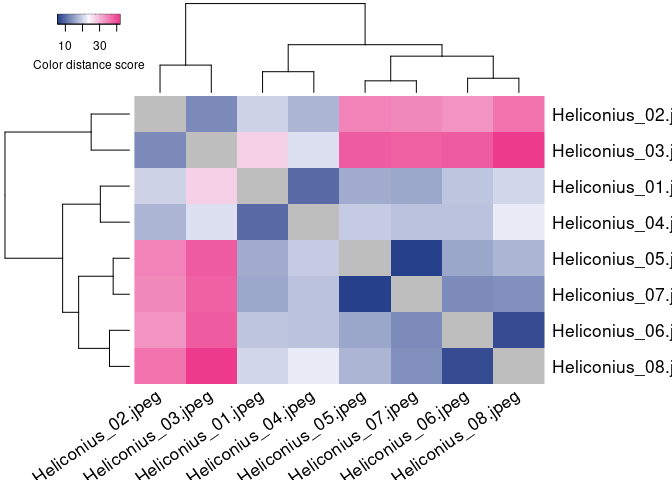The major difference is in your ability to interpret these results. Unlike in RGB space, where you have a well-defined maximum color distance using EMD (the cost of moving all pixels the farthest possible linear distance in a cube with sides of length 1 = $$\sqrt{3}$$), there is no absolute upper limit to the color distance in CIELab space. However, the results can still be interpreted relative to each other. You can also determine reasonable upper limits given a certain reference white, since you’re starting with RGB pixels, which can only occupy a subset of CIELab space.